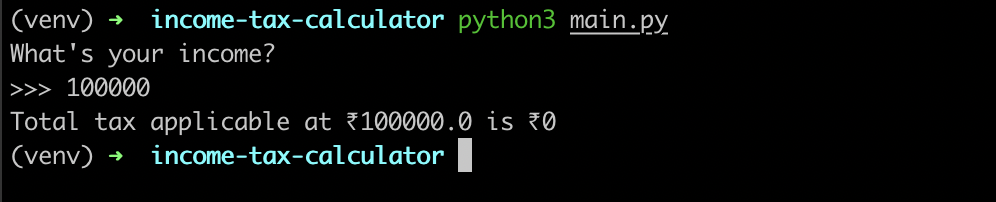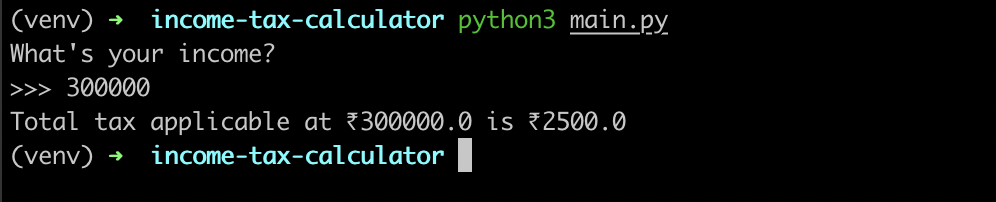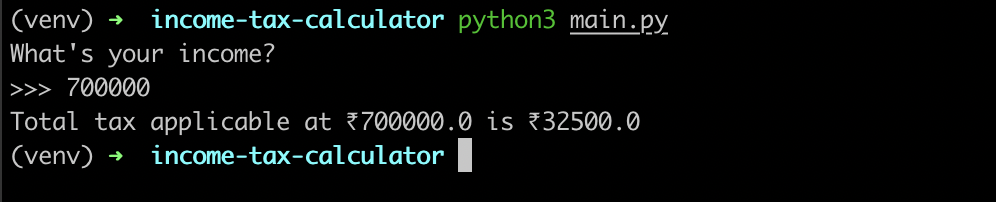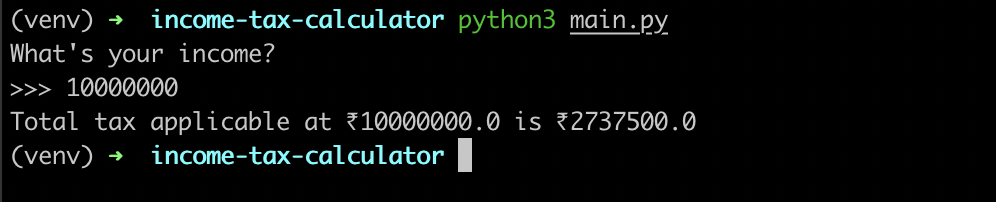Open in App
Not now

# Income Tax Calculator using Python

• Last Updated : 07 Nov, 2022

In this article, We’ll create a Python program that determines the income tax owed on a specific quantity of money. Please be informed that the income tax slabs are changed every year. Since income taxes vary from nation to nation, we have created a program that solely supports the Indian format and uses India as the country for tax calculation.

Tax slabs for AY 2022-23

AMOUNT                             INCOME TAX RATE
Up to ₹2,50,000                     0%
₹2,50,001 – ₹5,00,000           5% above ₹2,50,000
₹5,00,001 – ₹7,50,000           10% above ₹5,00,000 + ₹12,500
₹7,50,001 – ₹10,00,000         15% above ₹7,50,000 + ₹37,500
₹10,00,001 – ₹12,50,000       20% above ₹10,00,000 + ₹75,000
₹12,50,001 – ₹15,00,000       25% above ₹12,50,000 + ₹1,25,000
Above ₹15,00,001                  30% above ₹15,00,000 + ₹1,87,500

### Python Program to Calculate Income Tax

In this program, we will take annual income from the user as input after that we will pass that data to compare the Tax slab with different if-else conditions. After comparing we will calculate the tax and return it to that function to print the calculated Tax on that Income.

## Python3

 `def` `calculate(amount, percent):``    ``return` `(amount ``*` `percent) ``/` `100`` ` `def` `calculate_income_tax(total_income: ``                         ``float``) ``-``> ``float``:`` ` `    ``if` `total_income <``=` `250000``:``        ``return` `0``    ``elif` `total_income <``=` `500000``:``        ``return` `calculate(total_income ``-` `                         ``250000``, ``5``)``    ``elif` `total_income <``=` `750000``:``        ``return` `calculate(total_income ``-` `                         ``500000``, ``10``) ``+` `12500``    ``elif` `total_income <``=` `1000000``:``        ``return` `calculate(total_income ``-` `                         ``750000``, ``15``) ``+` `37500``    ``elif` `total_income <``=` `1250000``:``        ``return` `calculate(total_income ``-` `                         ``1000000``, ``20``) ``+` `75000``    ``elif` `total_income <``=` `1500000``:``        ``return` `calculate(total_income ``-` `                         ``1250000``, ``25``) ``+` `125000``    ``else``:``        ``return` `calculate(total_income ``-` `                         ``1500000``, ``30``) ``+` `187500`` ` ` ` `if` `__name__ ``=``=` `'__main__'``:``    ``total_income ``=` `float``(``input``("What's your \``                    ``annual income?\n>>> "))``    ``tax ``=` `calculate_income_tax(total_income)``    ``print``(f"Total tax applicable at \``                    ``₹{total_income} ``is` `₹{tax}")`

Input 1: When your income is ₹1,00,000 which is less than ₹2,50,000 then the tax should be ₹0

Output:Input 2: When the income is ₹3,00,000 which is more than ₹2,50,000 then the tax should be ₹2,500

Output:Input 3: When the income is ₹7,00,000 which is more than ₹5,00,000 then the tax should be ₹32,500

Output:Input 4: When your income is ₹1,00,00,000 which is more than ₹15,00,000 then the tax should be ₹27,37,500

Output:My Personal Notes arrow_drop_up